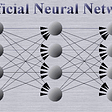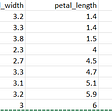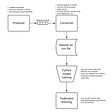# Term Frequency & Inverse Document Frequency : TF-IDF in NLP

Before we talk about TF-IDF I hope you’ve red my last article on Bag-of-Words if not then please read it then come back here.

In Bag-of-Words when we create a vector for any statement it is created in solid numbers i.e. non decimal numbers. Take an example of the following statement:

We’ve 11 columns and 3 rows and we are talking about the first statement i.e. ‘He wants to go USA’. The vector generated for this statement is ‘1–1–1–1–1–0–0–0–0–0–0’ for every present word in the sentence we got the number of times it is present. But this approach doesn’t give us the importance/weight for each word in the statement it is just telling us the number of time a word is present in the statement.

So to over come this problem we head towards TF-IDF.It does the same thing as BoW convert statements to vector but it also provides weight of each term i.e. word.

I would like to split them first we discuss TF & then IDF and at the end we multiply them with each other.

## Term Frequency (TF):

Term Frequency a simple definition is “how often a word occur in a document”. But there is a mathematical formula to find TF i.e. number of times a word occurred divided by total words.

But unlike BoW TF won’t create columns of unique words instead it creates columns of statement and rows of unique words.

Assume we have the following three statements the table for this will be created 3 columns and 9 rows.

We’ve found 9 unique words in three statements and when finding the TF for each statement what we do is let’s take example of first statement i.e. ‘John love to play poker’.

Total words in statement is 5 so our denominator will remain same for all the words in this statement column.

• ‘John’ its in our statement and only one time so 1/5. (1 = total time this words occurs in the statement and 5 is the total number of words in the statement)
• ‘loves’ its in our statement and only one time so 1/5
• ‘to’ its in our statement and only one time so 1/5
• ‘play’ its in our statement and only one time so 1/5
• ‘poker’ its in our statement and only one time so 1/5

Rest of the words are not in our statement thats why all of them will be 0/5.

Now let’s move forward with IDF.

## Inverse Document Frequency (IDF):

Unlike TF where or denominator was constant for each statement in IDF our nominator will remain constant where as the denominator will be changing for each word.

look at the IDF column and remember the formula no. of sentences / no. of sentences with that word. In this case we have three sentences so our nominator for IDF will be 3 and now 3 will be with no. of sentences that word (word for the row) is present in.

We can see ‘John’ all of our three sentences contain this words so IDF for ‘John’ is log(3/3). ‘loves’ appears in two sentences so IDF for it is log(3/2). ‘to’ appears in one sentence hence IDF log (3/1) and so on.

Now to generate the final vector for each statement we will need to multiply TF with IDF. And this time our words will generate the columns where as statements will generate rows.

The table will look like this:

Now to fill this table we will take column of each sentence and multiply it with column of IDF. Below is the example for it and will the values for each statement against each column of word.

The final vector for this sentence will be :

And the final TF-IDF vector for all three would be :

John is 0 for all three how ever it was present in all three sentence i.e. beacuse TF-IDF returns a vector based on weights and not the presence.

## Practical Implementation in Python :

To start first I will show you the entire code and then will explain it.

`from sklearn.feature_extraction.text import TfidfVectorizerimport pandas as pdstat1= "John loves to play poker"stat2 = "John is good boy"stat3 = "John loves horses"vectorizer = TfidfVectorizer()vectors = vectorizer.fit_transform([stat1,stat2,stat3])feature_names = vectorizer.get_feature_names()dense = vectors.todense()df = pd.DataFrame(dense, columns=feature_names)print(df)print(vectorizer.idf_)from sklearn.feature_extraction.text import TfidfVectorizerimport pandas as pd`

The first two lines we are importing two libraries one is ‘TfidfVectorizer’ from ‘sklearn.feature_extraction.text’ this helps us to create TF-IDF vectors from our text and other is ‘pandas’ this helps us with cvs files and data frames.

`stat1= "John loves to play poker"stat2 = "John is good boy"stat3 = "John loves horses"`

Next we created three statements the one we took as an example.

`vectorizer = TfidfVectorizer() #object of TfidfVectorizer()vectors = vectorizer.fit_transform([stat1,stat2,stat3])`

Later we created the object of ‘TfidfVectorizer()’ and then we provided the data to be vectorized and stored the values of vectorized data in a variable named vectors.

`feature_names = vectorizer.get_feature_names()dense = vectors.todense() #returns data in matrix form`

feature name is self explanatory we are just getting our feature names from the vector i.e the unique words and dense is the matrix form of our vectors we will pass it as rows in our data frame.

`df = pd.DataFrame(dense, columns=feature_names)`

We created a data frame passed ‘dense’ as rows and ‘feature_names’ as columns.

`print(df)print(vectorizer.idf_)`

Then we printed the data frame but also the ‘IDF’ (vectorize.idf_) values for our features because the data frame will be showing us the ‘TF-IDF’ values.

Now you must be wondering the values we calculated manually and the values returned by vectorizers are different. Yes! they are different but the meaning for our values and these values are same.

The difference occur because we did ‘Standard Tf-IDF’ and the ‘TFidfVectorizer’ uses some extra steps in my next article I’ll be describing the differences and you will be able to understand why our values are changed from the vectorizer values.

If I was able to explain this topic well and you liked my article do share it with your friends and follow me this really motivates me to write more and more tutorials.

--

--

--

## More from Umair Ishrat Khan

An energetic and motivated individual IOS developer/ Data Science Practitioner. Apart from computer science Martial arts interests me the most.

Love podcasts or audiobooks? Learn on the go with our new app.

## How to get started with the Diabetic Retinopathy project## Insights from EMNLP 2017## What is an Artificial Neural Network…?## Statistical Tests in Machine Learning## Decision Trees in Machine Learning## Part 2: Selecting the right weight initialization for your deep neural network.## Parallel and distributed machine learning## Resources to get started with Keras and it’s Applications## Umair Ishrat Khan

An energetic and motivated individual IOS developer/ Data Science Practitioner. Apart from computer science Martial arts interests me the most.

## Component of Natural Language Processing (NLP)## Lemmatization In Natural Language Processing — NLP## Performance Analysis of Text-Summary Generation Models Using ROUGE Score